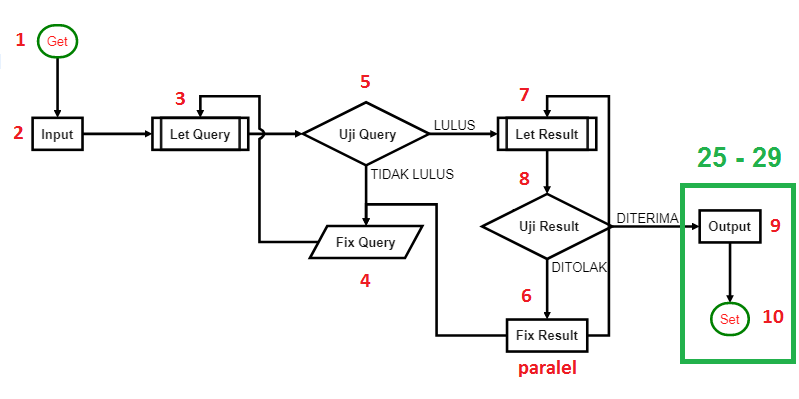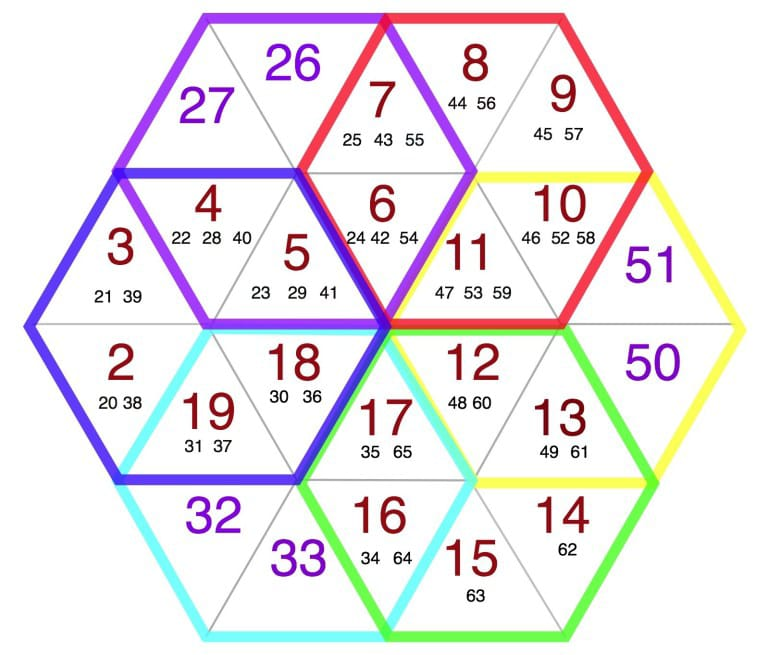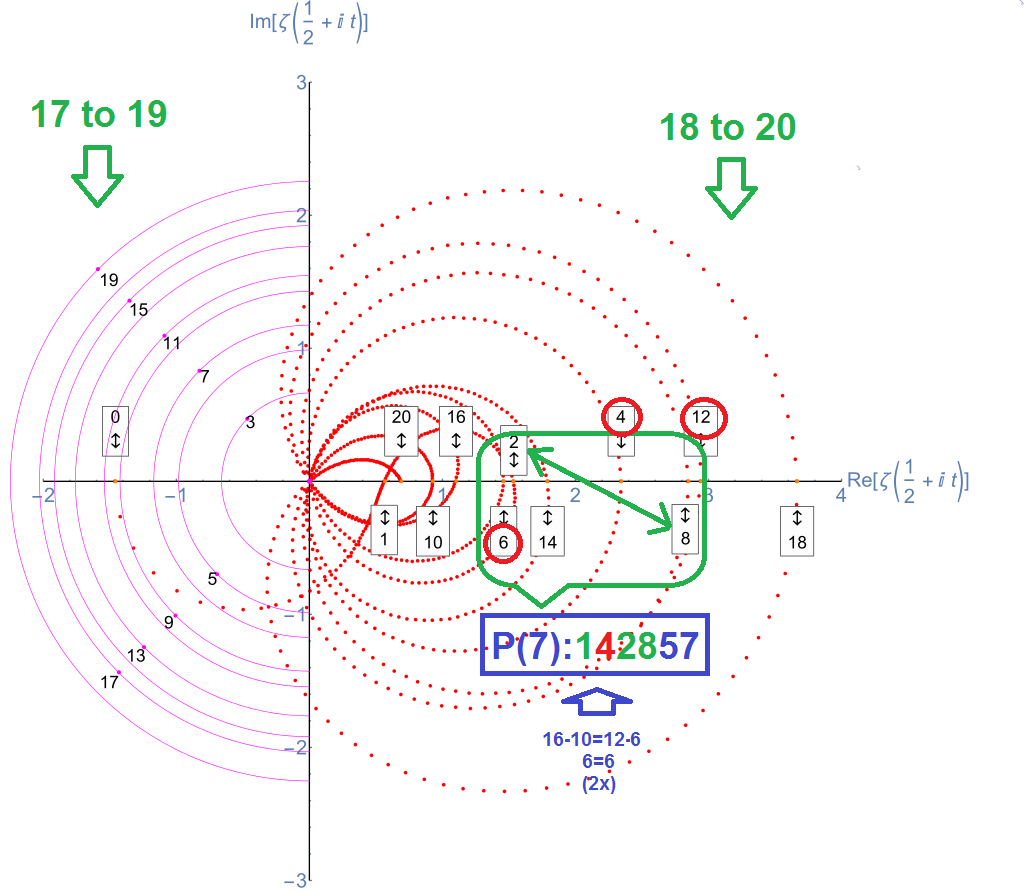# Team Work

||This repo is courtesy of The Quantum Project. Find all of them on The Project Map.|| |:—-|—-|—-:|

# Introduction

``````      <html> (29=10th prime)
|          <style>  - 5 ‹----
|                     Δ      |
3(29)‹------------ favicon - 2,3,5=π(5)
</style>          |
<body> (19)            |
<script> - 7 ‹----
</script>
</body
</html>
``````

<img src=https://user-images.githubusercontent.com/36441664/73946420-7cd3db80-4928-11ea-8cdf-1859c18a6c94.png>

## Tabulate Prime

Primes is formed by three (3) sets of twin prime pairs called The True Prime Pairs.

<img src=https://user-images.githubusercontent.com/36441664/90720864-ab703d00-e2e1-11ea-99c5-70405c78b6e0.jpg>

These primes are naturally distributed in three (3) forms of 12’s multiplication: 5+7=12, 11+13=24 and 17+19=36 where 12+24=36.

`````` \$True Prime Pairs:
(5,7), (11,13), (17,19)

layer|  i  |   f
-----+-----+---------
|  1  | 5
1  +-----+
|  2  | 7
-----+-----+---   36 » 6®
|  3  | 11
2  +-----+
|  4  | 13
-----+-----+---------
|  5  | 17
3  +-----+      36 » 6®
|  6  | 19
-----+-----+---------
``````

Now we are going to see what is the purpose. Let’s enter the journey of making 10n numbers becoming 10n primes by the Δ1 flowing within the prime pairs.

## 1st Step: Q(10, 30)

Let’s put this prime pairs (5, 7, 11, 13, 17,19) in reverse (19, 17, 13, 11, 7, 5) then assign them as a default condition. Consider the (12/2)th = 6th prime = 13 is formed as an hexagon cube called the Metatron’s Cube.

<img src=https://user-images.githubusercontent.com/36441664/74094157-4e642500-4b0f-11ea-89b4-eb8cd7d90411.png>

Then assign 19 as a set of 10 numbers that consist of 4 primes (2,3,5,7). Convert them to 10 primes up to 10th prime=29 then to 29 primes up to of (10th)th=29th prime=109. Now let’s put Δ1 to 13 as the central of Metraton.

``````  Tabulate Prime by Power of 10
loop(10) = π(10)-π(1) = 4-0 = 4
loop(100) = π(100)-π(10)-1th = 25-4-2 = 19
loop(1000) = π(1000) - π(100) - 10th = 168-25-29 = 114

--------------------+----+----+----+----+----+----+----+----+----+-----
True Prime Pairs Δ |  1 |  2 |  3 |  4 |  5 |  6 |  7 |  8 |  9 | Sum
====================+====+====+====+====+====+====+====+====+====+=====
π(10) 19           |  2 |  3 |  5 |  7 |  - |  - |  - |  - |  - | 4th  4 x Root
--------------------+----+----+----+----+----+----+----+----+----+-----
π(20) 17           | 11 | 13 | 17 | 19 |  - |  - |  - |  - |  - | 8th  4 x Twin
--------------------+----+----+----+----+----+----+----+----+----+-----
π(30) 13 → 12 (Δ1) | 23 | 29 |  - |  - |  - |  - |  - |  - |  - |10th
====================+====+====+====+====+====+====+====+====+====+===== 1st Twin
π(50) 11           | 31 | 37 | 41 |  - |  - |  - |  - |  - |  - |13th
--------------------+----+----+----+----+----+----+----+----+----+----- 2nd Twin
π(60) 7 → 19 (Δ12) | 43 | 47 | 53 | 59 |  - |  - |  - |  - |  - |17th
--------------------+----+----+----+----+----+----+----+----+----+----- 3rd Twin
π(80) 5 → 18 (Δ13) | 61 | 67 | 71 |  - |  - |  - |  - |  - |  - |20th
====================+====+====+====+====+====+====+====+====+====+===== 4th Twin
π(00) 0 → 18+13+12 | 73 | 79 | 83 | 89 | 97 | 101| 103| 107| 109|29th
====================+====+====+====+====+====+====+====+====+====+=====
Δ                                                         Δ
12+13+(18+18)+13+12  ← 36th-Δ1=151-1=150=100+2x(13+12)  ←  30th = 113 = 114-1
``````

See how those primes reacted to compensate the Δ1 by spreading the gap in to a bunch of Δ’s starting from the prime number 7.

<img src=https://user-images.githubusercontent.com/36441664/74990717-97549b80-5476-11ea-82d1-2d03f37fa695.png>

## 2nd Step: Q(30, 36)

In the sense of this Δ1 flowing, there will be really hard to cope its algorithm with a such of formula. The way that we might take is assigning the flowing of the π(10)=4 to π(100)=25 and π(1000)=168 above in to a flowchart diagrams.

<img src=https://user-images.githubusercontent.com/36441664/128732737-81762604-0ae0-4a90-b5a8-30921cf46efb.png>

See that this flows starting from π(10) and finalize by pairing of Δ12. Let’s draw 12 of flow arrows in such a way where they have interconnection with 10 objects. So here we can get the idea of 10 number becoming 10 primes.This flowchart is designed base on the behaviour of the primes number that flows uniformly within an hexagon chart called The Prime Hexagon. Here you might see that the number of 10 is the same direction with 19 while 10+19=29=10th prime.

<img src=https://user-images.githubusercontent.com/36441664/105412087-d0892300-5c66-11eb-9c3e-19d8652b478c.png>

By checking all of the loops there is nothing similar to this phenomena. It seems like all the numbers are actually set to let this term happen. So this chart is considered sufficient enought to be used to explain about the true power of this Δ1 flowing:

Let’s start by get in touch with the (30, 36)th as the result of Δ1 shown on the above tabulation in more detail. Then take a look with the behaviour of prime flows within The Prime Hexagon as shown below.Take a note that the next 10 number after 19 right before the 30 which are 20 up to 29 is in laid on the second rows of 17 while 17’s Cell has 35+65=100=10².

You may see also that it is clearly showing the 30th Prime of 113=114-1 is routed to 36th Prime of 151=150+1 while both of the 30 and 36 are exactly laid on the 18’s Cell:

## 3rd Step: Q(36, 114)

See that from this 17’s to18’s it goes finally to the 19’s Cells as Δ1 and return to the 2’ Cells, 3’ Cells and so on. Therefore all of the numbers that involved in the hexagon can be tabulated by Δ1=(19 vs 18) Loops and they are end exactly at 114 on 19’s!

``````       |         1st (Form)          |         2nd (Route)         |         3rd (Channel)         |
-----+----+----+----+----+----+----+----+----+----+----+----+----+----+----+-----+-----+----+----+
150 | 151| 152| 153| 154| 155| 156| 157| 158| 159| 160| 161| 162| 163| 164| 165 | 166 | 167| 168|
=====+====+====+====+====+====+====+====+====+====+====+====+====+====+====+=====+=====+====+====+
Δ1 | 19 |  - | 31 | 37 |  - |  - |  - |  - |  - |  - |  - |  - |  - |  - | 103 |  -  |  - |  - |
+----+----+----+----+----+----+----+----+----+----+----+----+----+----+-----+-----+----+----+
Δ2 | 20 | 26|  - | 38 |  - |  - |  - |  - |  - | 74|  - |  - |  - | 98| 104|  -  |  - |  - |
-----+----+----+----+----+----+----+----+----+----+----+----+----+----+----+-----+-----+----+----+
Δ3 | 21 | 27|  - | 39 |  - |  - |  - |  - |  - | 75|  - |  - |  - | 99| 105|  -  |  - |  - |
+----+----+----+----+----+----+----+----+----+----+----+----+----+----+-----+-----+----+----+
Δ4 | 22 | 28 |  - | 40 |  - |  - |  - |  - |  - | 76 |  - |  - |  - |100 |  -  |  -  |  - |  - |
-----+----+----+----+----+----+----+----+----+----+----+----+----+----+----+-----+-----+----+----+
Δ5 | 23 | 29 |  - | 41 |  - |  - |  - |  - |  - | 77 |  - |  - |  - |101 |  -  |  -  |  - |  - |
+----+----+----+----+----+----+----+----+----+----+----+----+----+----+-----+-----+----+----+
Δ6 | 24 |  - |  - | 42 |  - | 54 |  - |  - | 72 | 78 |  - | 90 | 96 |  - |  -  |  -  |  - | 114|<- 6th row
=====+====+====+====+====+====+====+====+====+====+====+====+====+====+====+=====+=====+====+====+
Δ7 | 25 |  - |  - | 43 |  - | 55 |  - |  - | 73 | 79 |  - | 91 | 97 |  - |  -  |  -  |  - |  - |
+----+----+----+----+----+----+----+----+----+----+----+----+----+----+-----+-----+----+----+
Δ8 |  - |  - |  - | 44 |  - | 56 |  - |  - |  - | 80 |  - | 92 |  - |  - |  -  |  -  |  - |  - |
-----+----+----+----+----+----+----+----+----+----+----+----+----+----+----+-----+-----+----+----+
Δ9 |  - |  - |  - | 45 |  - | 57 |  - |  - |  - | 81 |  - | 93 |  - |  - |  -  |  -  |  - |  - |
+----+----+----+----+----+----+----+----+----+----+----+----+----+----+-----+-----+----+----+
Δ10 |  - |  - |  - | 46 | 52 | 58 |  - | 70 |  - | 82 | 88 | 94 |  - |  - |  -  |  -  | 112|  - |
-----+----+----+----+----+----+----+----+----+----+----+----+----+----+----+-----+-----+----+----+
Δ11 |  - |  - |  - | 47 | 53 | 59 |  - | 71 |  - | 83 | 89 | 95 |  - |  - |  -  |  -  | 113|  - |
+----+----+----+----+----+----+----+----+----+----+----+----+----+----+-----+-----+----+----+
Δ12 |  - |  - |  - | 48 |  - | 60 | 66 |  - |  - | 84 |  - |  - |  - |  - |  -  | 108 |  - |  - |
=====+====+====+====+====+====+====+====+====+====+====+====+====+====+====+=====+=====+====+====+
Δ13 |  - |  - |  - | 49 |  - | 61 | 67 |  - |  - | 85 |  - |  - |  - |  - |  -  | 109 |  - |  - |
+----+----+----+----+----+----+----+----+----+----+----+----+----+----+-----+-----+----+----+
Δ14 |  - |  - | 32| 50|  - | 62 | 68|  - |  - | 86|  - |  - |  - |  - |  -  | 110|  - |  - |
-----+----+----+----+----+----+----+----+----+----+----+----+----+----+----+-----+-----+----+----+
Δ15 |  - |  - | 33| 51|  - | 63 | 69|  - |  - | 87|  - |  - |  - |  - |  -  | 111|  - |  - |
+----+----+----+----+----+----+----+----+----+----+----+----+----+----+-----+-----+----+----+
Δ16 |  - |  - | 34 |  - |  - | 64 |  - |  - |  - |  - |  - |  - |  - | -  | 106 |  -  |  - |  - |
-----+----+----+----+----+----+----+----+----+----+----+----+----+----+----+-----+-----+----+----+
Δ17 |  - |  - | 35 |  - |  - | 65 |  - |  - |  - |  - |  - |  - |  - | -  | 107 |  -  |  - |  - |
+----+----+----+----+----+----+----+----+----+----+----+----+----+----+-----+-----+----+----+
Δ18 |  - | 30 | 36 |  - |  - |  - |  - |  - |  - |  - |  - |  - | -  |102 |   - |  -  |  - |  - | <- ∑=168
=====+====+====+====+====+====+====+====+====+====+====+====+====+====+====+=====+=====+====+====+
1  |  2 |  3 |  4 |  5 |  6 |  7 |  8 |  9 | 10 | 11 | 12 | 13 | 14 | 15 |  16 |  17 | 18 | 19 |
-----+----+----+----+----+----+----+----+----+----+----+----+----+----+----+-----+-----+----+----+
|       Δ    Δ                |                     Φ12     |       Δ                     Δ |
113  150                                   114-25           557                  1000

Note:
- The marked number with  are outside of group Δ18 vs loop(100) = 19
- Number 114 located on 6th row vs 19th column whereas 114th prime = 619

Reference:
https://github.com/chetabahana/hexagon/pull/2
https://www.hexspin.com/defining-the-prime-hexagon/
``````

See that out of 19 the number 89 is located precissely at the of Δ11 within the end of 12’s that goes to 13’s Cell. So this is related to the Metratron’s Cube. This configuration leads to a Cyclic in the Loops as followings:

• Injection goes by 114-π(100)=89 on Δ11 from 102 to 114-1 or 113
• The 113 is 30th prime where 30, 36 and 102 are laid in the end of rows
• The total of 30+36+102 is exactly 168 which is π(1000)
• The 36th prime which is 151, is reinjected by 151-1 or 150. This 150 is carrying a delta of Δ18 with 168
• The Δ18 brings the 30th and 36th cycled back to 102th thus consequently it goes to the 114th prime
• The 114th prime which is carrying the loop(1000) is reinjected by 619-1 or 618 on the same spot.

You may agree that all of this algorithm seems like a primes orchestra. By sourcing and studying of many references, the closest behaviour of the crossing on above primes flowing is found with the wave shown on Δ1=(19 vs 18) Loops as below.This Δ(19 vs 18) scenario will duplicate the loops of 618 as π(89²) of 1000 Primes. Thus its behaviour will return to 168 Primes of π(1000) out of the adjacent scheme.

So the further process would always continously become the same algorithm.